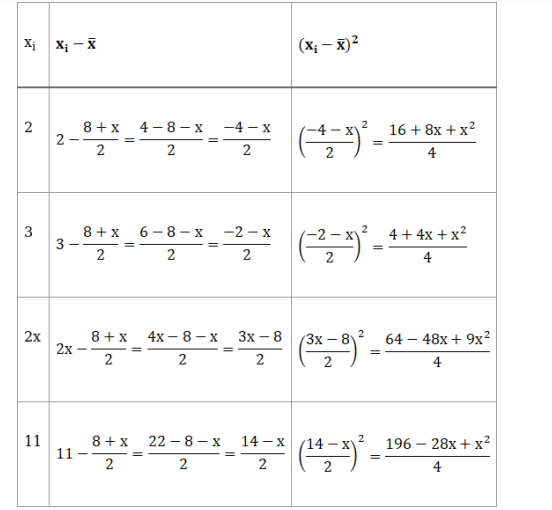Deepak Scored 45->99%ile with Bounce Back Crack Course. You can do it too!

# If the standard deviation of the numbers 2, 3, 2x, 11 is 3.5,

Question:

If the standard deviation of the numbers 2, 3, 2x, 11 is 3.5, calculate the possible values of x.

Solution:

Given: Standard Deviation, $\sigma=3.5$

and Numbers are 2, 3, 2x, 11

We know that,

Mean $(\overline{\mathrm{x}})=\frac{\text { Sum of observations }}{\text { Total number of observations }}$

$=\frac{2+3+2 x+11}{4}$

$=\frac{16+2 x}{4}$

$\overline{\mathrm{x}}=\frac{8+\mathrm{x}}{2}$Variance, $\sigma^{2}=\frac{1}{\mathrm{n}} \sum\left(\mathrm{x}_{\mathrm{i}}-\overline{\mathrm{x}}\right)^{2}$

$(3.5)^{2}=\frac{1}{4}\left[\frac{16+8 x+x^{2}}{4}+\frac{4+4 x+x^{2}}{4}+\frac{64-48 x+9 x^{2}}{4}+\frac{9-6 x+x^{2}}{4}\right]$

\begin{aligned} \Rightarrow 12.25=& \frac{1}{16}\left[16+8 x+x^{2}+4+4 x+x^{2}+64-48 x+9 x^{2}+196-28 x\right.\\ &\left.+x^{2}\right] \end{aligned}

$\Rightarrow 12.25 \times 16=280-64 x+12 x^{2}$

$\Rightarrow 196=280-64 x+12 x^{2}$

$\Rightarrow 12 x^{2}-64 x+280-196=0$

$\Rightarrow 12 x^{2}-64 x+84=0$

$\Rightarrow 3 x^{2}-16 x+21=0$

$\Rightarrow 3 x^{2}-9 x-7 x+21=0$

$\Rightarrow 3 x(x-3)-7(x-3)=0$

$\Rightarrow(3 x-7)(x-3)=0$

Putting both the factors equal to 0, we get

$3 x-7=0$ and $x-3=0$

$\Rightarrow 3 x=7$ and $x=3$

$\Rightarrow x=\frac{7}{3}$

Hence, the possible values of $x$ are $\frac{7}{3} \& 3$